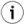## 11.3.Data Types

Virtuoso/PL supports the regular SQL scalar data types as well as user-defined-types (UDTs). UDTs and structures can be composed of data types or classes made from any hosted language such C# or Java. Local temporary tables are not supported by the present Virtuoso but may be added in the future.

Memory management is automatic. Parameters, cursors and intermediate results are automatically allocated and freed.

A parameter or variable can be of any data type a column can. Variables are however typed at run time, so that the declared type is mostly for documentation. The declared types also affect how interactive SQL shows certain values.

There is a special `vector` construct, declared as type `ANY` , that can be used as an array. Vectors must be instantiated using the `vector()` function, optionally containing initial elements as a comma-separated list, and can be increased in size using the `vector_concat()` . Elements of a vector are read and changed using the `aref()` and `aset()` functions respectively. A special notation can be used as a short-hand for accessing the elements of a vector one-level deep only. The notation is by using the variable with the index in square brackets. Hence, `aref(vec, 1)` is the same as `vec` . The following example reveal more:

Example11.1.Using Vectors

Simple excerpt showing how to instantiate a vector.

```declare vec1, vec2, vec3 any;

vec1 := vector();  -- simple empty vector
vec2 := vector('a', 'b', 1, 2);  -- vector of mixed types
vec3 := vector(vector('a', 'b'), vector(1, 2));  -- vector of vectors
```

Second-level elements of vec3 cannot be referenced as vec3.

Here is the code for a simple VSP page that shows how vectors can be used.

```<html>
<body>
<?vsp
declare vec1 any;
declare i integer;

vec1 := vector();

i := 0;
while ( i <= 5 ) {
vec1 := vector_concat(vec1, vector(i * 5));
i := i + 1;
}

http('displaying the contents using aref<br>');
i := 0;
while ( i <= 5 ) {
http_value(aref(vec1, i)); http('<br>');
i := i + 1;
}

http('changing the values using aset<br>');
i := 0;
while ( i <= 5 ) {
aset(vec1, i, i * 10);
i := i + 1;
}

http('displaying the contents using [] notation<br>');
i := 0;
while ( i <= 5 ) {
http_value(vec1[i]); http('<br>');
i := i + 1;
}

http('changing the values using [] notation<br>');
i := 0;
while ( i <= 5 ) {
vec1[i] := i * 15;
i := i + 1;
}

http('displaying the contents using [] notation again<br>');
i := 0;
while ( i <= 5 ) {
http_value(vec1[i]); http('<br>');
i := i + 1;
}
?>
</body>
</html>
```

Which produces the following output:

```loading up the vector using vector_concat...
displaying the contents using aref
0
5
10
15
20
25
changing the values using aset
displaying the contents using [] notation
0
10
20
30
40
50
changing the values using [] notation
displaying the contents using [] notation again
0
15
30
45
60
75
```

See Also: